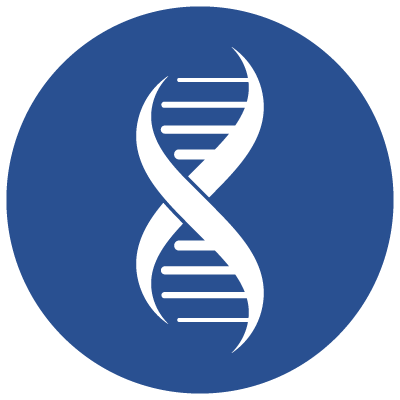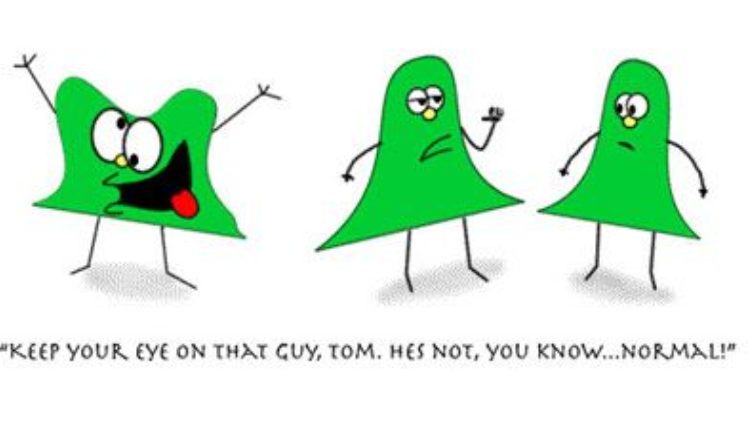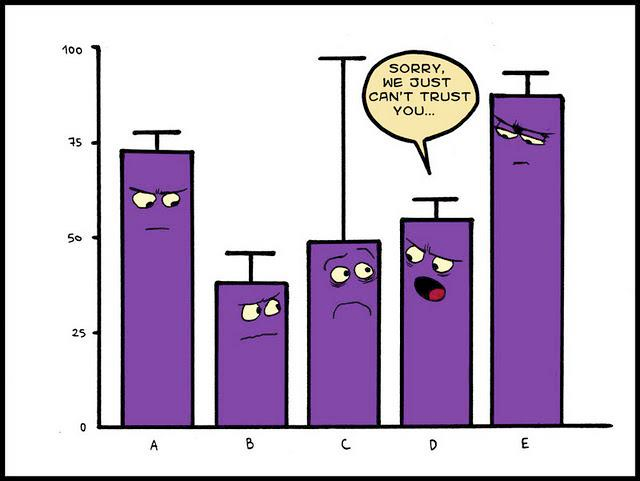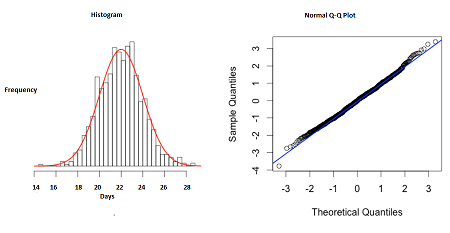# Statistics For ProgrammersDid you know that while analysing data in statistics we test for normality? One easy way to check for normality is to check the mean, median and mode of the data. If all these are relatively equal then we assume the data is “approximate normal”. We can confirm this either using formal statistical tests or we can use visual inspection methods to check for normality. Do you know how to test them?We use Kolmogorov-Smirnov (KS) Test or Shapiro-Wilk (SW) test to confirm the normality of the data.
If the p-values from these tests are greater than 0.05, then we assume normality of the data.

How to visually inspect your data for normality?We can inspect them by plotting a histogram or a normal quantile-quantile plot (Q-Q Plot).

Histograms are a commonly used graphical method to determine the distribution of a continuous variable. If the graph is approximately
bell-shaped and symmetric about the mean, we can assume normality.

Normal Quantile-Quantile Plot (Q-Q Plot): Plot the quantiles of a variable’s distribution against the quantiles of the normal distribution. If the data is normal, the points should form an approximate straight line. See examples of a approximate normal histogram and Q-Q here: